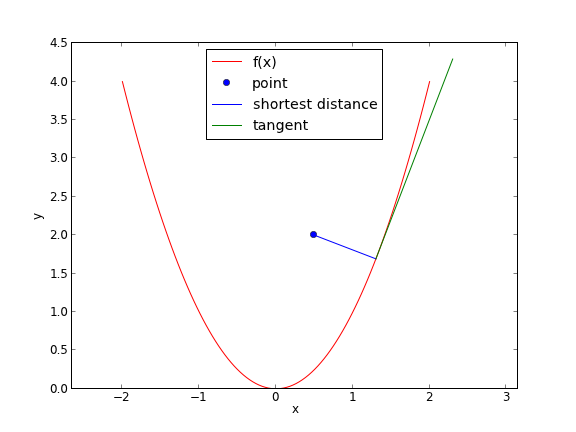## Find the minimum distance from a point to a curve.

| categories: optimization | tags:

A problem that can be cast as a constrained minimization problem is to find the minimum distance from a point to a curve. Suppose we have $$f(x) = x^2$$, and the point (0.5, 2). what is the minimum distance from that point to $$f(x)$$?

import numpy as np
import matplotlib.pyplot as plt
from scipy.optimize import fmin_cobyla

P = (0.5, 2)

def f(x):
return x**2

def objective(X):
x,y = X
return np.sqrt((x - P)**2 + (y - P)**2)

def c1(X):
x,y = X
return f(x) - y

X = fmin_cobyla(objective, x0=[0.5,0.5], cons=[c1])

print 'The minimum distance is {0:1.2f}'.format(objective(X))

# Verify the vector to this point is normal to the tangent of the curve
# position vector from curve to point
v1 = np.array(P) - np.array(X)
# position vector
v2 = np.array([1, 2.0 * X])
print 'dot(v1, v2) = ',np.dot(v1, v2)

x = np.linspace(-2, 2, 100)

plt.plot(x, f(x), 'r-', label='f(x)')
plt.plot(P, P, 'bo', label='point')
plt.plot([P, X], [P, X], 'b-', label='shortest distance')
plt.plot([X, X + 1], [X, X + 2.0 * X], 'g-', label='tangent')
plt.axis('equal')
plt.xlabel('x')
plt.ylabel('y')
plt.legend(loc='best')
plt.savefig('images/min-dist-p-func.png')

The minimum distance is 0.86
dot(v1, v2) =  0.000336477214214

Normal return from subroutine COBYLA

NFVALS =   44   F = 8.579598E-01    MAXCV = 0.000000E+00
X = 1.300793E+00   1.692061E+00In the code above, we demonstrate that the point we find on the curve that minimizes the distance satisfies the property that a vector from that point to our other point is normal to the tangent of the curve at that point. This is shown by the fact that the dot product of the two vectors is very close to zero. It is not zero because of the accuracy criteria that is used to stop the minimization is not high enough.